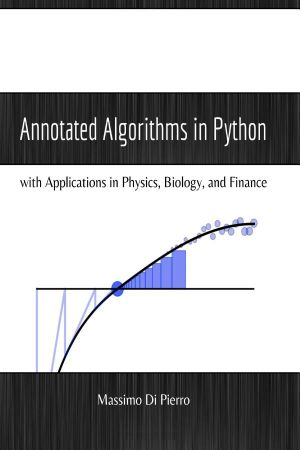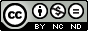# Annotated Algorithms in Python

## With applications in Physics, Biology, and Finance

by Massimo Di PierroDescriptionTable of ContentsDetailsHashtagsReport an issue

### Book Description

This book is assembled from lectures given by the author over a period of 10 years at the School of Computing of DePaul University. The lectures cover multiple classes, including Analysis and Design of Algorithms, Scientific Computing, Monte Carlo Simulations, and Parallel Algorithms. These lectures teach the core knowledge required by any scientist interested in numerical algorithms and by students interested in computational finance.

The algorithms you will learn can be applied to different disciplines. Throughout history, it is not uncommon that an algorithm invented by a physicist would find application in, for example, biology or finance.

This open book is licensed under a Creative Commons License (CC BY-NC-ND). You can download Annotated Algorithms in Python ebook for free in PDF format (4.6 MB).

### Table of Contents

Chapter 1
Introduction

Main Ideas

About Python

Book Structure

Book Software
Chapter 2
Overview of the Python Language

About Python

Types of variables

Python control flow statements

Classes

File input/output

How to import modules
Chapter 3
Theory of Algorithms

Order of growth of algorithms

Recurrence relations

Types of algorithms

Timing algorithms

Data structures

Tree algorithms

Graph algorithms

Greedy algorithms

Artificial intelligence and machine learning

Long and infinite loops
Chapter 4
Numerical Algorithms

Well-posed and stable problems

Approximations and error analysis

Standard strategies

Linear algebra

Sparse matrix inversion

Solvers for nonlinear equations

Optimization in one dimension

Functions of many variables

Nonlinear fitting

Integration

Fourier transforms

Differential equations
Chapter 5
Probability and Statistics

Probability

Combinatorics and discrete random variables
Chapter 6
Random Numbers and Distributions

Randomness, determinism, chaos and order

Real randomness

Entropy generators

Pseudo-randomness

Parallel generators and independent sequences

Generating random numbers from a given distribution

Probability distributions for continuous random variables

Resampling

Binning
Chapter 7
Monte Carlo Simulations

Introduction

Error analysis and the bootstrap method

A general purpose Monte Carlo engine

Monte Carlo integration

Stochastic, Markov, Wiener, and processes

Option pricing

Markov chain Monte Carlo (MCMC) and Metropolis

Simulated annealing
Chapter 8
Parallel Algorithms

Parallel architectures

Parallel metrics

Message passing

mpi4py

Master-Worker and Map-Reduce

pyOpenCL
Appendix A
Math Review and Notation

### Book Details

Subject
Computer Science
Publisher
Experts4Solutions
Published
2013
Pages
388
Edition
2
Language
English
ISBN13 Digital
9780991160402
ISBN10 Digital
0991160401
PDF Size
4.6 MB
License### Related BooksClassic Computer Science Problems in Python deepens your knowledge of problem solving techniques from the realm of computer science by challenging you with time-tested scenarios, exercises, and algorithms. As you work through examples in search, clustering, graphs, and more, you'll remember important things you've forgotten and discover classic sol...Extremely versatile and popular among developers, Python is a good general-purpose language that can be used in a variety of applications. For those with an understanding of English, Python is a very humanreadable programming language, allowing for quick comprehension. Because Python supports multiple styles including scripting and object-oriented ...Invent Your Own Computer Games with Python teaches you how to program in the Python language. Each chapter gives you the complete source code for a new game, and then teaches the programming concepts from the examples. Games include Guess the Number, Hangman, Tic Tac Toe, and Reversi. This book also has an introduction to making games with 2D graph...Puzzle-based learning is an active learning technique. With code puzzles, you will learn faster, smarter, and better. Coffee Break Python Slicing is all about growing your Python expertise - one coffee at a time. The focus lies on the important slicing technique to access consecutive data ranges. Understanding slicing thoroughly is crucial for y...Learn how graph algorithms can help you leverage relationships within your data to develop intelligent solutions and enhance your machine learning models. With this practical guide, developers and data scientists will discover how graph analytics deliver value, whether they're used for building dynamic network models or forecasting real-world behav...This book offers a concise and gentle introduction to finite element programming in Python based on the popular FEniCS software library. Using a series of examples, including the Poisson equation, the equations of linear elasticity, the incompressible Navier - Stokes equations, and systems of nonlinear advection - diffusion - reaction equations, it...